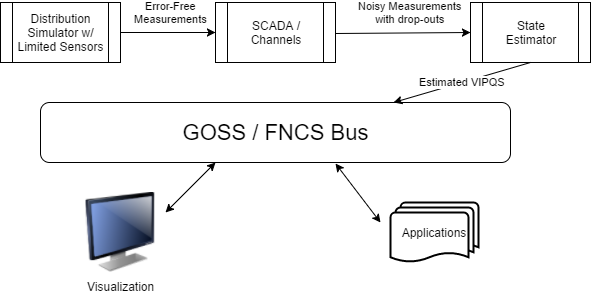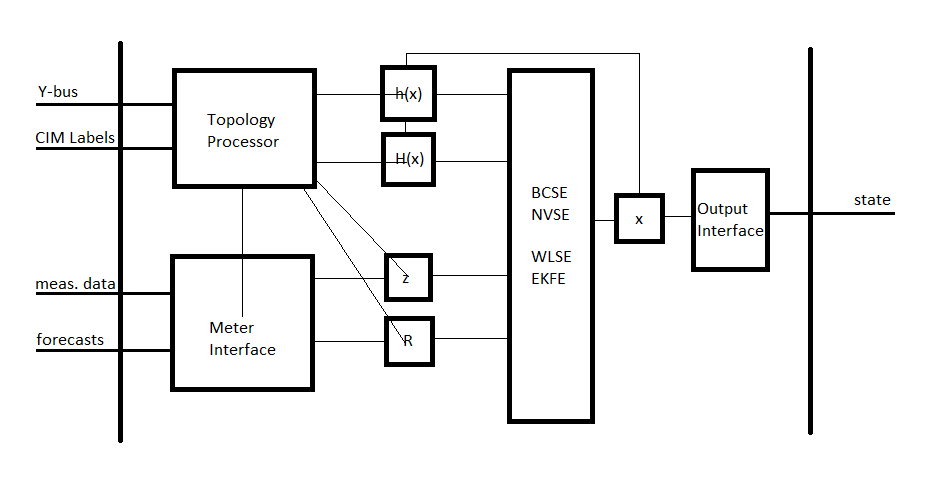# Integrated Applications¶

## Volt-var Optimization (VVO)¶

The sample VVO application is a Python implementation of a heuristic method that PNNL has investigated before [CIT3], [CIT4], [CIT5]. There are more advanced VVO methods that will be implemented in future work.

## Visualization¶

We have created a web-based visualization of the sample VVO application. The visualization displays the topology of the IEEE 8500-Node system as an interactive graph. Capacitors and regulators are highlighted in the graph and displayed alongside tables with current values for capacitor status (OPEN or CLOSED), regulator voltage, and feeder power.

## State Estimator Service¶

Given a perfect and complete set of voltage magnitude and angle measurements, along with a detailed and accurate power system model, one could calculate the real power, or any other electrical variable of interest, anywhere in the system. In practice, measurements have errors, time delays, and may even be missing. State estimation refers to the process of minimizing the errors and filling in gaps . One state estimation method is called “weighted least squares”, and it’s analogous to drawing the best-fit line through a set of scattered points. Other methods may perform better . Also, on distribution systems, it may be better to estimate branch currents instead of node voltages, but the principle is the same. In GridAPPS-D, the visualizations and applications ought to use the best available state estimator outputs, instead of raw SCADA values, for both accuracy and consistency. Therefore, the state estimator is not an application but a service in GridAPPS-D, sitting between emulated SCADA and the GOSS bus.Figure 1: The state estimator processes noisy and incomplete measurements, then posting estimated voltage (V), current (I), real power (P), reactive power (Q) and switch status (S) values onto the GridAPPS-D message / data bus.

In Figure 1, the power system model (upper left) will include a limited number of sensors, corresponding to actual voltage and current transformers, line post sensors, wireless sensors, etc. In some scenarios, smart meters can also be sensors. Each such sensor will have different performance characteristics (e.g. precision, accuracy, sampling rate). Distribution systems typically do not have enough sensors to make the system observable, so there will be measurement gaps in the topology. The state estimator might fill these gaps with interpolation and graph-tracing methods on the power system model.

The supervisory control and data acquisition (SCADA) system in Figure 1 introduces more errors and failure points. Eventually, GridAPPS-D may simulate these impacts by federating ns-3 as a co-simulator. Until then, a placeholder module could be used to insert variable errors, time delays and dropouts in each measurement, whether due to sensor characteristics or the communication system. The output represents data as it would come into an operations center, and feeds the state estimator. Internally, the data flows between simulator, SCADA and state estimator might be implemented with FNCS, but this is an implementation detail. The state estimator will provide two outputs to the GOSS bus used by all GridAPPS-D applications:

1. At a time step configured by the platform, publish the best-estimate VIPQS values wherever sensors actually exist in the model, with quality attributes that still have to be established. Sensor locations delineate circuit segments, and note that all VIPQS values will be estimated at the boundaries, even if the sensor measures only V or I, for example.
2. Upon request by another application or service, publish the estimated VIPQS values for all nodes and components in the model, even at locations where no sensors exist. A variant is to publish the estimates only for selected nodes and components.

As indicated in Figure 1, other applications need to obtain estimated VIPQS values from the GOSS bus. Switch open/close states are a special case; they might be considered known values, but in practice the switch state is a measurement, which could lead to topology errors in the model. For GridAPPS-D, switch state estimates need to be a point of emphasis. Given that most distribution systems lack redundant measurements, It would be possible for an application to query these VIPQS values directly from the simulator or SCADA, bypassing the state estimator, but this is “cheating” in most situations. However, in the application development process, idealized VIPQS values could be obtained through a combination of two methods:

1. Add more sensors to the power system model
2. Set the sensor and channel errors to zero

Because the sensor outputs in GridAPPS-D come from a power flow solution that enforces Kirchhoff’s Laws, the state estimator will produce ideally accurate values whenever the sensor and channel errors have been specified to be zero. The state estimator may still exhibit interpolation errors between sensor locations, but that is readily mitigated for testing purposes by adding more sensors.

With reference to RC1, the visualization and VVO applications should now subscribe to VIPQS values from the state estimator, not from the distribution simulator. They may also use or display quality metrics on the estimated values.

### Design Objectives¶

State estimation is widely used in transmission system operations but is less common in distribution system operations due to a relatively limited value in traditional distribution systems, additional computational complexity, and a lack of sensors. Advanced distribution management platforms like GridAPPS-D provide access to model and sensor data that can be leveraged to overcome barriers to adoption and open the door to distribution system state estimators that are fast and accurate enough to be useful in utility operations.

A distribution system state estimator computes the most likely state given a set of present and/or past measurements. The full state of a distribution system consists of either the full set of complex bus voltages or the full set of complex branch currents; given the system model (admittance matrix), the remaining system parameters can be computed given the full system state.

### Use Cases¶

• Assist power factor optimization: Utility objective is unity power-factor at the substation.
• Assist voltage optimization (planning): Utility objective is 1 p.u. voltage at last house primary.
• Real-time state estimation for advanced applications: applications can access the state estimate at a sufficient resolution to capture e.g. insolation variation caused by clouds.

### Algorithms¶

State estimation uses system model information to produce an estimate of the state vector x given a measurement vector z. The measurement vector is related to the state vector and an error vector by the measurement function, which may be non-linear.

$z = h(x) + e$

Multiple formulations of the distribution system state estimation problem are possible:

1. Node Voltage State Estimation (NVSE): The state vector consists of node voltage magnitudes and angles for each node in the system (one reference angle can be eliminated from the state vector). This formulation of the state estimation problem is general to any topology and it is the standard for transmission system state estimation.
2. Branch Current State Estimation (BCSE): Radial topology and assumptions about shunt losses create a linear formulation of the state estimation problem. The state vector contains branch currents and, for a fully-constrained problem, requires one state per load, which can be less than the number of branches in the system.

Different algorithms provide different advantages for distribution system state estimation. A subset of the state estimation algorithms below will be used to achieve these goals.

1. Weighted Least Squares Estimation (WLSE): a concurrent set of measurements are used to find a state vector that minimizes the weighted least squares objective function. The algorithm is memoryless with respect to previous solutions and measurements should be synchronized.
2. Kalman Filter Estimation (KFE) and Extended Kalman Filter Estimation (EKFE): The Kalman filter provides a mechanism to consider past state estimates alongside present measurements. This provides additional noise rejection and allows asynchronous measurements can be considered individually. KFE is appropriate for linear BCSE and EKFE is compatible with nonlinear NVSE.
3. Unscented Kalman Filter Estimation (UKFE): The unscented transform estimates the expected value and variance of the system state by observing the system outputs for inputs spanning the full dimensionality of the measurement space. Again, the Kalman filter provides a mechanism to consider past estimates.

### TRL¶

The state estimator application will provide the capability to estimate the full system state using asynchronous measurement data. In addition a model order reduction technique will be implemented to greatly speed up the state estimation computation and to reduce the dependence on forecast-based pseudo-measurements. A paper (Reduced-Order State Estimation for Power Distribution Systems with Sparse Sensing) is targeted for IEEE Transactions on Power Systems.

### Architecture¶

The state estimation service is being developed in c++. A modern c++ implementation allows the application to adapt to an evolving interface. The program architecture is shown below.Topology Processor: initializes the measurement function and its Jacobian and determines the size of the measurement vector, the measurement covariance matrix, and the state vector.

Meter Interface: updates the measurement vector and the measurement covariance matrix as new measurement data comes available.

State Estimator: performs the state estimation operation according to the specified algorithm.

Output Interface: formats the state vector and any implicit states as an output stream.

### Inputs¶

Upon initialization, the topology processor will receive the Y-bus from the GridLAB-D service and will query contextual information and sensor locations from the CIM database.

Periodic measurement data, including any forecasts to be used a pseudo-measurements will be required as inputs.

A “terminate” command from the platform will end the state estimation process.

### Outputs¶

The output will include the full system state (node voltages and/or branch currents TBD).

### Testing and Validation¶

Evaluation metrics

• State Error: compare state estimation output to “true” system state.
• Accuracy over baseline: compare state error of state estimator to state error of a QSTS load-flow model.
• Execution Time

Scenarios

• Full sensor deployment: verify that the true system state can be reproduced.
• Sparse sensor deployment: verify that the state estimator performs better than a QSTS load-flow model.
• Breaker trip: verify that switch state can be detected even when it is reported incorrectly.
• Bad sensor detection: verify that a sensor that is producing bad data can be identified.
• Dependent application support: verify that the state estimator can support e.g. the VVO application.
• Fault: for a radial system, determine the nearest common bus from multiple emulated customer calls.

### Operating/Running¶

The state estimator will execute the topology processor at initialization and will enter a stat estimation loop. The state estimation loop will exit and the process will end upon receiving a ‘terminate’ command from the platform.

At initialization, a configuration file will be read for:

• State estimation mode (state vector and algorithm) selection
• Normalized residual threshold for bad measurement / sensor detection